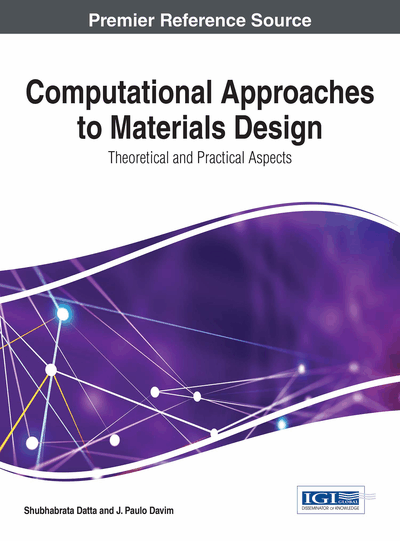# Applications of Feature Selection and Regression Techniques in Materials Design: A Tutorial

Partha Dey (Academy of Technology, Adisaptagram, Hooghly, India), Joe Bible (Eunice Kennedy Shriver National Institute of Child Health and Human Development, USA), Swati Dey (Indian Institute of Engineering, Science and Technology Shibpur, India) and Somnath Datta (University of Florida, USA)
DOI: 10.4018/978-1-5225-0290-6.ch008

## Abstract

Feature selection is considered as an important preprocessing step to data mining and soft computing, whereas regression is a collection of methods to optimally assess the signal from a noisy output. Both seek to arrive at the dependence and relation between different attributes and a target material property. In the present chapter a flock of regression and feature selection techniques are discussed, and the kind of results that can be obtained with each of them has been illustrated with the help of a dataset on steel. The different methods are capable of abstracting data in different forms, thus revealing hidden knowledge from different perspectives. Choosing the most appropriate method depends on the application at hand and the kind of objective that one is looking for.
Chapter Preview
Top

## Regression Techniques Based On Statistical Learning

The most classical regression technique is the ordinary least squares (OLS), which works well when the number of predictors is small, the correlation between the predictors is weak and their contributions to the conditional mean response are linear and additive. Departure from these assumptions would require considering more sophisticated methods. As for example, when the number of predictors is greater than the number of observations, OLS is not applicable. The aforementioned task of addressing model creation in the presence of a large number of descriptors can be accomplished via the implementation of one or several applicable regression techniques; Principle Component Regression (PCR), Partial Least Squares (PLS), Sparse Partial Least Squares (SPLS), Least Absolute Shrinkage and Selection Operator (Lasso), Ridge Regression and Elastic Net are examples of such techniques. The first three belong to a class of techniques referred to as latent factor techniques, the last three a class referred to as regularized regression techniques. Both are capable of handling a large number of descriptors with the distinction being that latent factor regression accomplishes this end by performing the analysis using a set of derived components (latent factors) whereas regularization does so by allowing descriptors to contribute disproportionately to the analysis.

Principle Component Analysis constructs a set of vectors (principle components), each of which describes the projection of each descriptor onto a p-dimensional space. PCR performs regression analysis using this set of principle components. PLS unlike PCR takes into account the response (target variable/property) as well as the response variables in constructing the latent factors used in analysis. PLS derives the latent factors in a way that maximizes the covariance between the latent factors and the target vector. SPLS introduces a sparsity parameter into PLS analysis which adds another dimension to the investigation.

Lasso can be characterized in terms of a penalized least squares analysis, through the introduction of a penalty on the absolute magnitude of the coefficients. Placing a penalty on the absolute magnitude of the coefficients has a desirable side effect in that it allows descriptors to discretely enter the model as well as contribute disproportionately. Ridge regression accomplished a similar end by introducing a penalty on the size of the squared magnitude of the coefficients, the quadratic penalty allows disproportionate contribution but not discrete selection. Elastic Net places both an absolute and quadratic penalty on the size of the coefficients.

## Complete Chapter List

Search this Book:
Reset